# How To Prepare Unit On Capacitor For NSEP

###### ByRamraj Saini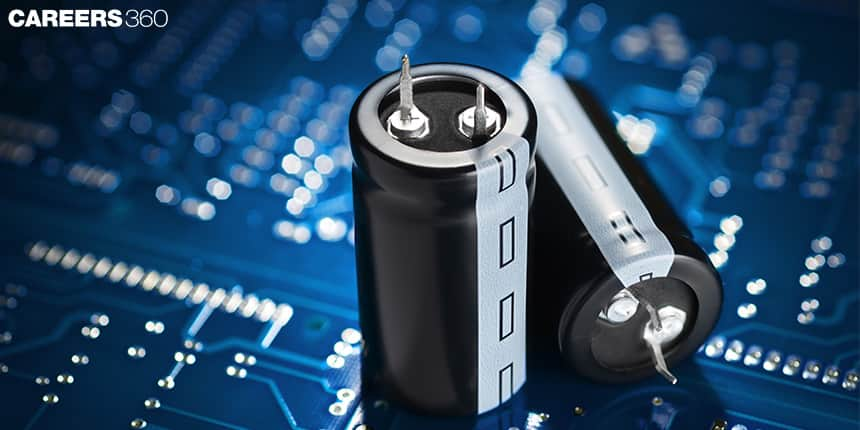###### Synopsis

Two or three questions from the concept of Capacitors are asked in the National Standard Examination in Physics (NSEP). But, that doesn’t mean you can ignore the chapter. This article contains some important concepts, formulas, and previous year's questions that can help students to do well in the exam.###### Synopsis

Two or three questions from the concept of Capacitors are asked in the National Standard Examination in Physics (NSEP). But, that doesn’t mean you can ignore the chapter. This article contains some important concepts, formulas, and previous year's questions that can help students to do well in the exam.

A capacitor is a device that stores electrical energy in the form of an electrostatic field. It is a system of two conductors closely separated by an insulator. The capacitor is a fundamental component of most electrical devices therefore it is used in a wide range of industries.

The concepts of capacitors are mixed with the concepts of electrostatics, current electricity, the magnetic effect of current, electromagnetic induction, and alternating current. Here in this article, we will discuss the important concepts of capacitors and also provide some previous year's questions of NSEP to practice the concepts. Interested students can also refer to the Class 12 NCERT chapter on capacitors to practice more problems on capacitors.

Also, Read| How To Prepare NSEP For Ray Optics

## Important Concepts In Capacitors

1. The charge on a plate is known as the charge on the capacitor

2. The electric field outside the capacitor is zero and inside the capacitor is constant which is equal to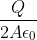3. The direction of an electric field is from the plate on which the positive charge is to the plate on which the negative charge is.

4. If the potential difference between the plates is V and the charge on the capacitor is Q then the relation between the charge and the potential difference is given by Q= CV In this relation C is the capacitance, Q is the charge on the plates and V is a potential difference.

There are different types of capacitors like parallel plate capacitors, spherical capacitors, cylindrical capacitors, and many more. Here mostly we will discuss parallel plate capacitors.

The capacitance of the parallel plate capacitor is given by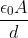. In this relation A is the surface area of the plate, epsilon is the permittivity of free space, and d is the distance between the plates.

## Equivalent Capacitance

If two or more capacitors are combined then we can calculate the equivalent capacitance. Mostly are two types of combination i.e series combination and parallel combination.

1. the equivalent capacitance of two capacitors C1 and C2 connected in In series combination is given by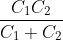.

2. If there are more than two capacitors C1, C2, C3…. are connected in series combination then equivalent capacitance C is given by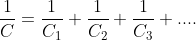.

3. The equivalent capacitance of capacitors C1, C2, C3…. which are connected in parallel combination is given by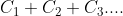4. The important concept to calculate equivalent capacitance is to connect the complex system with a V-volt battery and find the charge Q flow from the battery. The ratio of charge Q and Voltage V is the equivalent capacitance of the complex system.

5. Equivalence Capatience when the capacitor is filled with dielectrics K is given by KC where C is the capacitance of the capacitor without the dielectric.

## Energy Stored In Capacitor

Energy is the capacity to do work. Energy stored in the capacitor is given by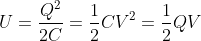## NSEP Previous Year Questions From Capacitor

Q-1 (NSEP 2018-19)

The total capacitance between points A and B in the arrangement shown below is: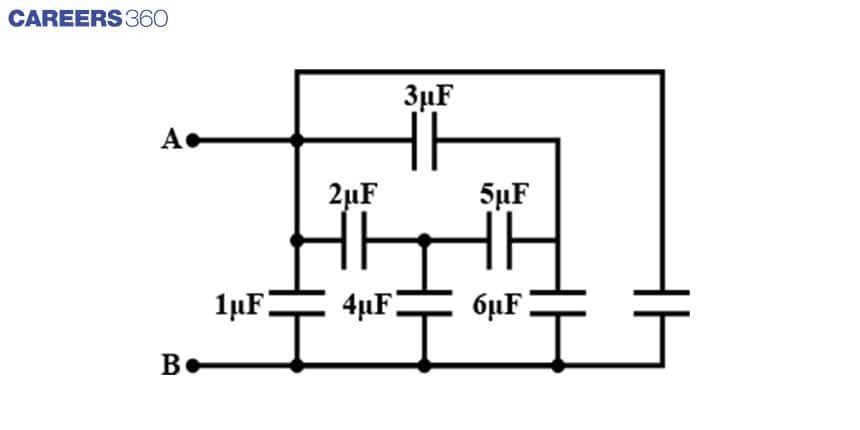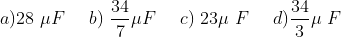Q-2 (NSEP 2017-18)

Consider a parallel plate capacitor. When half of the space between the plates is filled with some dielectric material of dielectric constant K as shown in Fig. (1) below, the capacitance is C₁. However, if the same dielectric material fills half the space as shown in Fig. (2), the capacitance is C2. Therefore, the ratio C₁: C₂ is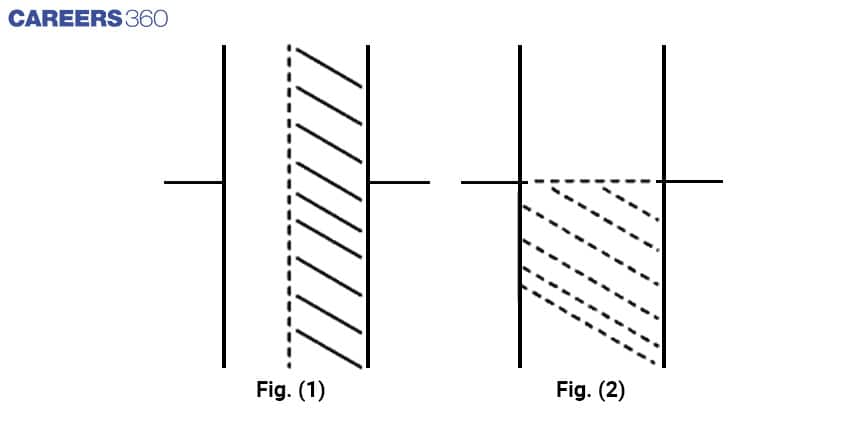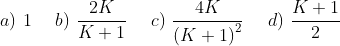Q-3 (NSEP 2016-17)

A simple laboratory power supply consists of a transformer, bridge rectifier, and a filter capacitor. It drives a suitable load. If due to some reason one of the diodes in the rectifier circuit becomes open, then

(A) The output voltage of the power supply falls to zero.

(B) The output voltage of the power supply decreases to some nonzero value

(C) AC ripple in the output increases.

(D) Ripple frequency decreases.

Also, Read| How To Prepare Magnetic Effects Of Current

Q-4 (NSEP 2015-16)

In the circuit shown below the switch is closed at t = 0. For 0 < t < R (C1 + C2), the current I1 in the capacitor C1 in terms of total current I is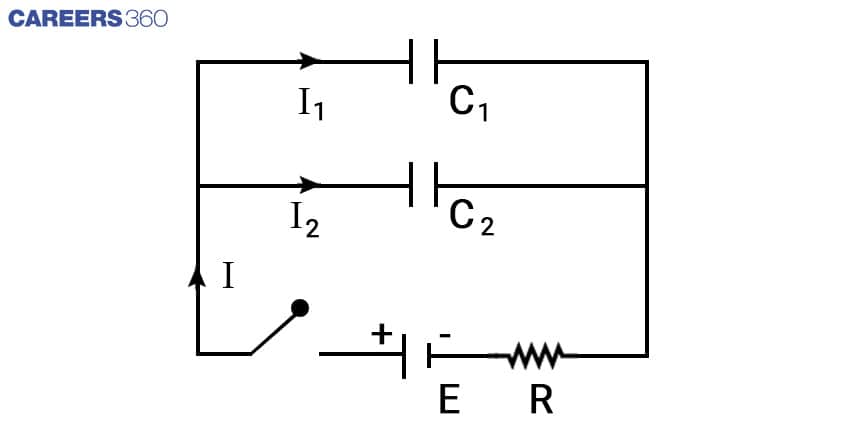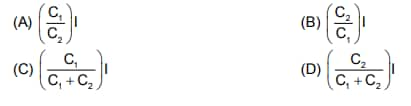## NSEP Study Materials For Capacitor

• NCERT Class 12 Physics chapter Capacitor

• NCERT Exemplar problems from Capacitor

• NSEP previous year's question papers

Also, Read| How to Prepare Electrostatics for NSEP

• Technology
• Physics
• Electromagnetism
• Electricity
• Electrical engineering
• Physical quantities
• Metrology
• Quantity
• Electronics
• Electronic engineering

## Careers360 helping shape your Career for a better tomorrow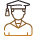#### 250M+

Students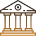#### 30,000+

Colleges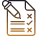#### 500+

Exams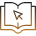E-Books